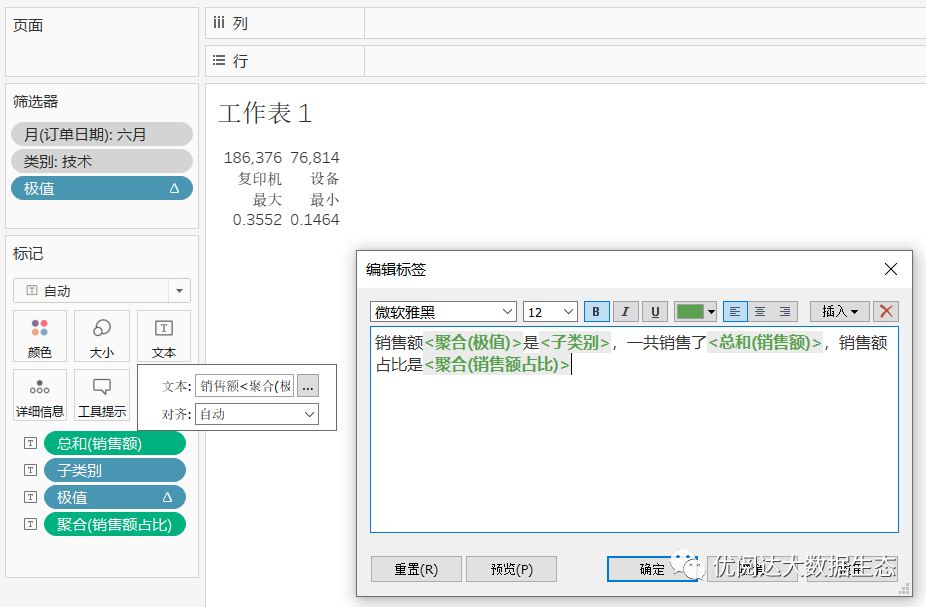published: 2021-09-10 17:25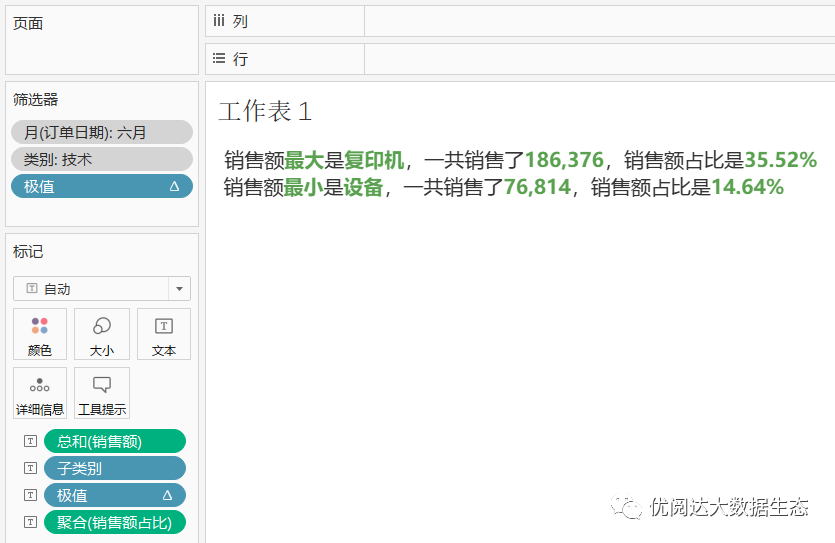1. 创建文本表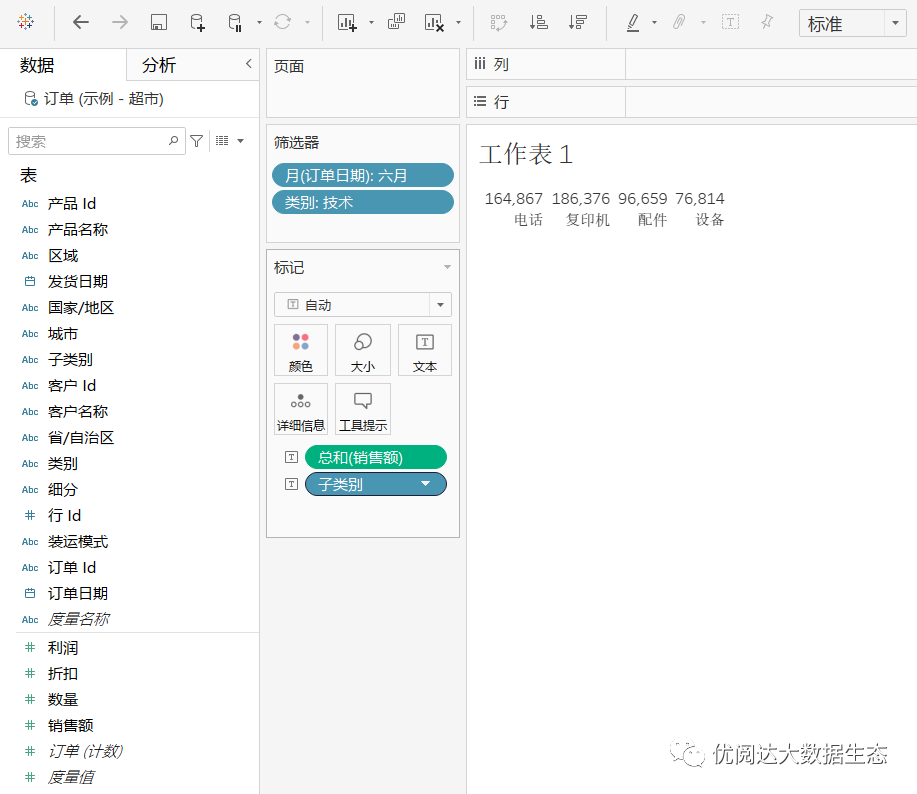2. 创建计算字段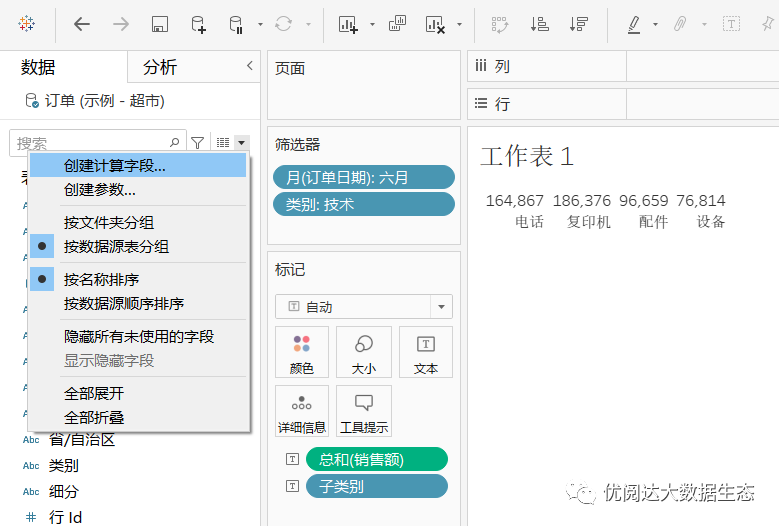➤ 计算字段：极值，键入函数

IF RANK(SUM(销售额),'asc')=1

then '最小'

ELSEIF RANK(SUM(销售额),'desc')=1

then '最大'

End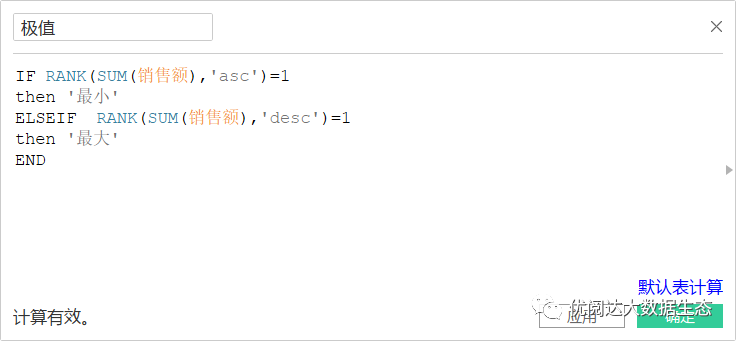➤ 计算字段：销售额占比，键入函数

SUM([销售额])/MIN({SUM([销售额])})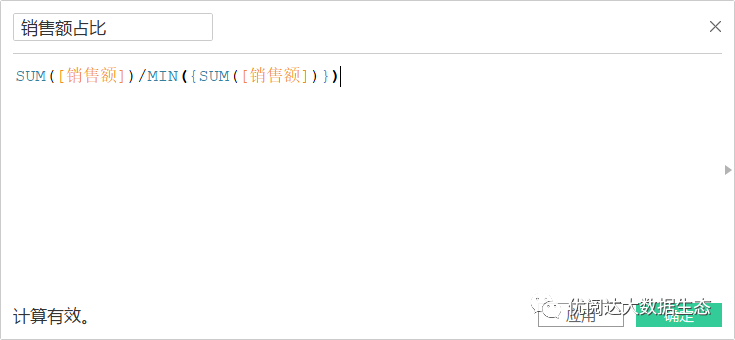Tips：这里的MIN({SUM([销售额])}) ，相当于MIN({fixed ：SUM([销售额])})，为了不受筛选器影响看总销售额，而这个MIN没有具体的含义，就相当于一种聚合的方式，也可以写成MAX({SUM([销售额])})

3. 设置极值筛选器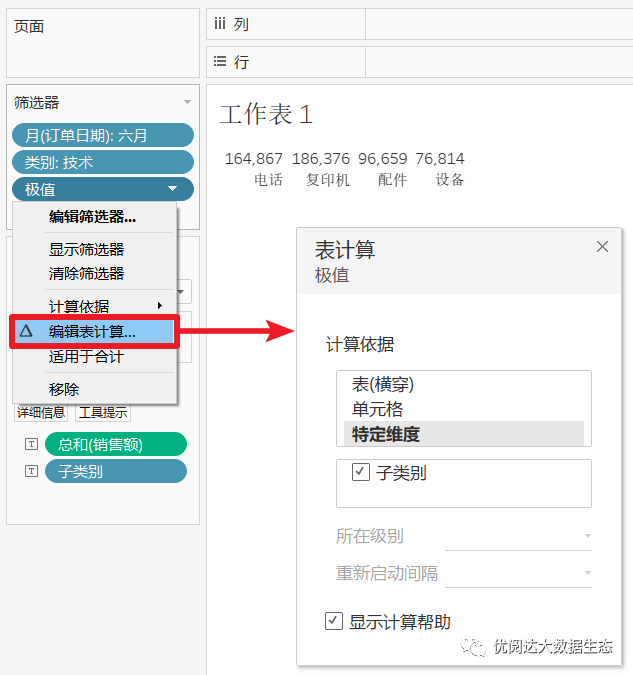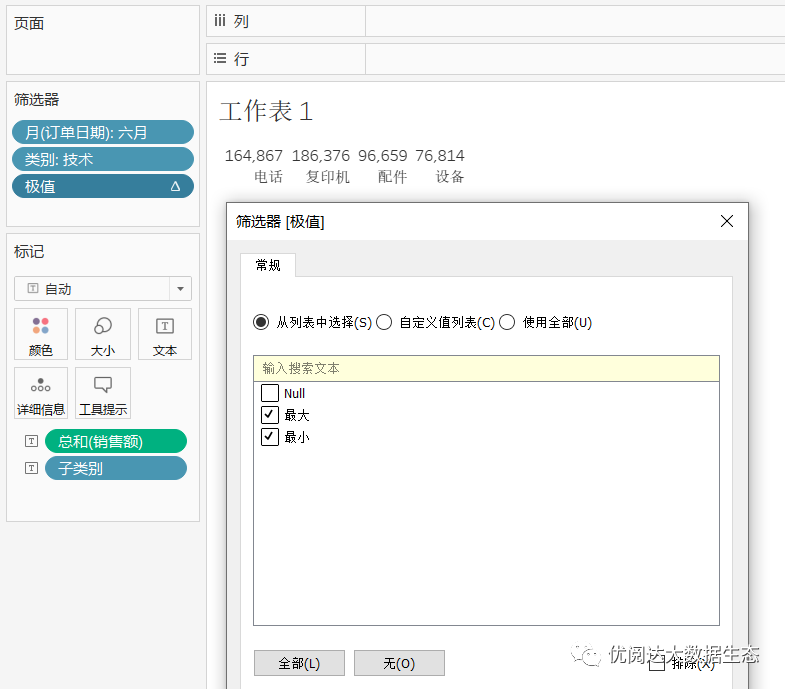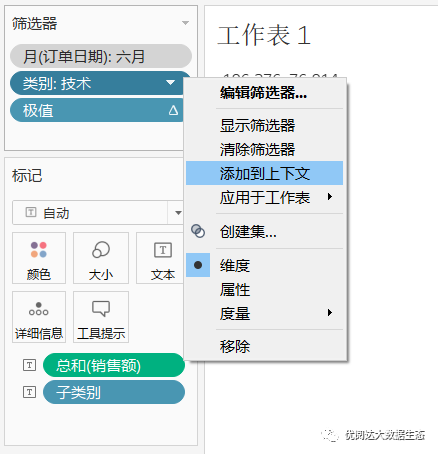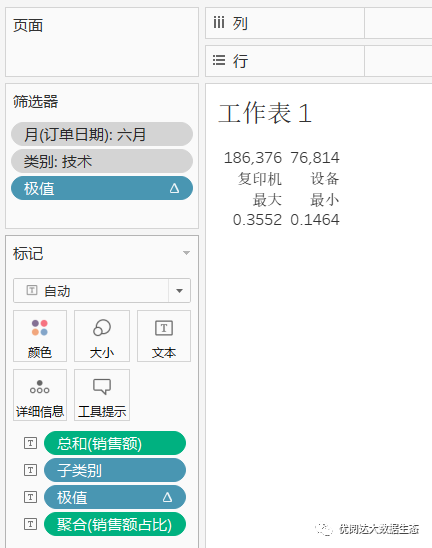4. 编辑文本

Tips：文本中直接展现出来的文字需要手动输入，再把字段拖放到文字后面，再调整相应的字体大小和颜色即可。